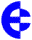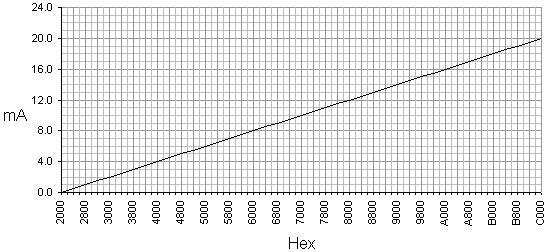Current/Hex value conversion Current values are relayed by Elpro in hexi-decimal (referred to as 'hex'). The range of 4-20mA is represented by the hex range 4000h to C000h. The calculation is I = 4 + (16 x ((T - 4000h) ÷ 8000h)) where I is the current in mA and T is the hex value being transmitted. This equates to 800h per mA. To make life simple the following graph provides a guide of current to hex value relationship. The range 2000h to C000h has been included as there are inputs and outputs capable of 0mA.Useful figures: 0mA = 2000h 4mA = 4000h 12mA = 8000h (midrange) 20mA = C000h (full range) © 14.08.00